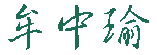Chung-Yu Mou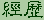Experience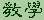Teaching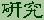Research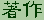Publication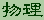Physics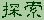##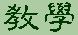Teaching

• 奈米科技與量子的魔力

• 打水漂兒的簡化模型
• 雲的形成
• 家用電表的簡化模型
• 有質量彈簧的振盪
• ### 電磁學(I)(II) 2014-2015

• 課程大綱(Syllabus)
• 筆記

• Introduction and Vector Analysis
• Electrostatics: description and basic properties
• Electric potentials and methods of their evaluation
• Electric fields in matter
• Magnetostatitics
• Magnetic fields in matter
• Electrodynamics
• Conservation Laws
• Electromagnetic waves
• Special relativity and electrodynamics
• Potentials and fields
• 習題 (I)

• 第3版與第4版習題對照表
• 習題 (II)

### Condensed Matter Physics (凝態物理) (I) 2019 Fall

• 課程大綱(Syllabus)
• Lecture note (筆記)

• Introduction, Crystal Structures, and Theory of Scattering in Conensed Matter
• General Consideration of Scattering Experiments
• Beyond Crystalline State
• Mechanical and dynamical properties
• Phonons in liquids/gases and the need for the 2nd quantization
• Phenomena of Phase Transition and Ginzburg-Landau Theory
• Defects, liquid-solid transition and 2D melting
• Electronic Structure - Free Electron Gas
• Waves in periodic structures
• Electronic Band Theory
• Semiclassical electron dynamics
• 投影片

• Quasicrystals
• Fullerenes
• 習題

• Homework 1
• Homework 2
• Homework 3
• Homework 4
• Homework 5
• ### 凝態物理(II) 2011 Spring

筆記

• Waves in non-crystalline structures and localization
• Methods in Quantum Many-Particle Theory (I)
• Methods in Quantum Many-Particle Theory (II)
• Linear Response Theory and Experimental Measurements
• 習題

• Homework 1
• ### 應用數學(I) 2011 Spring

• 課程大綱(Syllabus)
• ### 理論力學 (I) 2017 Fall

• 課程大綱(Syllabus)
• 筆記

• Kinematics -- Mathematical description of motion
• Newtonian Mechanics
• Oscillations and Chaotic Motions
• Principle of Virtual Work
• Hamilton's principle and the Lagragian/Hamiltonian dynamics
• Gravitation and Central-Force Motion
• 習題

• Homework 1
• Homework 2
• Homework 3
• Homework 4
• Homework 5
• Homework 6
• Homework 7
• Homework 8
• Homework 9
• ### 理論力學 (I) 2008 Fall

• 課程大綱(Syllabus)
• ### 理論力學 (II) 2009 Spring

• 課程大綱(Syllabus)
• 筆記

• Special Theory of Relativity
• Dynamics of a system of particles - scattering problems
• Motion in non-inertial frame
• Rigid-body rotations
• Coupled oscillations and elastic waves

### 量子物理 - Quantum Physics (I)(II), 2019-2020

• 課程大綱(Syllabus)
• 教科書大綱(Syllabus)
• 量子物理 (I) 習題

• Homework 1
• Homework 2
• Homework 3
• Homework 4
• Homework 5
• Homework 6
• Homework 7
• 筆記

量子物理 (I)

• The emergence of Quantum Physics
• Wave-particle duality, probability, and the Schrodinger equation
• Eigenvalues, Eigenfuncitons, and the expansion postulate
• One dimensional potentials
• The general structure of wave mechanics
• Prove of uncertainty relation
• N-particle systems
• The Schrodinger equation in 3D (I)
• 量子物理 (II)

• The Schrodinger equation in 3D (II): Angular momentum and H atom
• Space quantization and eigensates of angular momentum
• The hydrogen atom
• The interaction of charged particles with the electromagnetic fields
• Spin and its discovery
• Time independent perturbation theory
• The real hydrogen atom
• Ground state of multi-electron atoms and periodic table
• Interaction of EM waves and atoms/molecules
• Molecules and their energy spectrum
• Slides (投影片)

• Diffraction of electrons through double-slit (電子雙狹縫實驗)
• 相速度,群速度,與信號速度
• 量子物理 (II)

統計力學 (II) - Statistical Mechanics (II), Fall 2012

筆記

### 量子力學 - Quantum Mechanics (I)(II), 2018-2019

Lecture notes (筆記)

Quantum Mechanics (I)

• Foundations and Dirac notations
• Simple 1D systems
• Classical Limit and WKB approximation
• The Ucertainty Principle
• The Path Integral Formulation
• Harmonic Oscillator
• Identical Particles
• Symmetries and their consequences
• Quantum Mechanics (II)

• Theory of angular momentum & rotations
• Approximate methods
• Introduction to scattering theory
• Introduction to relativistic quantum mechanics
• Slides (投影片)

• Introduction
• Diffraction of electrons through double-slit (電子雙狹縫實驗)
• Usage of Quantum State: Quantum Cryptography, Grover algorithm and etc.
• Homework set (習題) (I)

• Homework 1
• Homework 2
• Homework 3
• Homework 4
• Homework 5
• Homework 6
• Homework set (習題) (II)

• Homework 1
• Homework 2
• Homework 3
• Homework 4
• Homework 5
• Homework 6